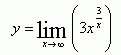SEARCH HOMEMath Central Quandaries & QueriesQuestion from Teodora, a student: What is the limit of 3.x^3/x as x approaches +infinity?Hi Teodora,

If I understand your question, you can let y be the limit you are trying to find:Here is a hint: Take the natural log of both sides of this expression. Then work with the expression on the right to find the natural log of the limit you are trying to find. By applying logarithm rules to the new expression on the right you can rewrite it so that you don't have to deal directly with a function of x to the power of a function of x.

You may have to use L'Hospital's rule if you don't know the limit of ln(x)/x as x -> infinity.

Remember: In the end you will end up with the natural log of the limit you were trying to find, not the actual limit you want.

HaleyMath Central is supported by the University of Regina and The Pacific Institute for the Mathematical Sciences.